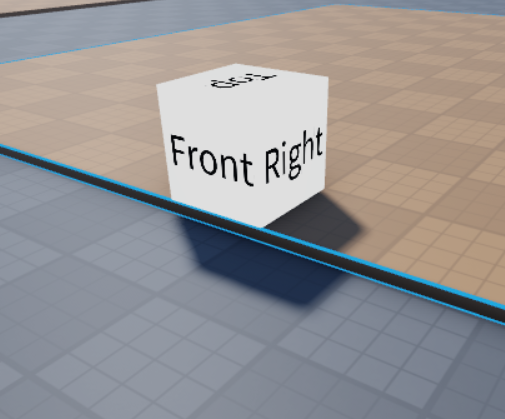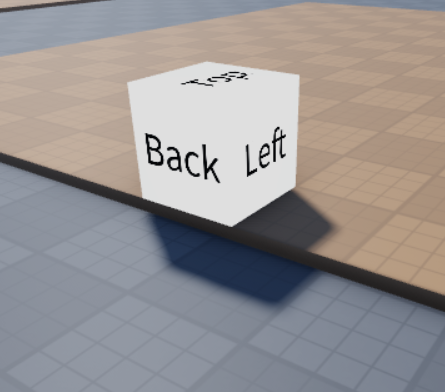Hello! I am working on a sandbox tycoon plot saving system and have encountered one issue with it. The system works flawlessly except for the fact it adds on the plots rotation to the models each time you load the data back in. For example, say the plots rotation is 180 degrees on the y axis, you place down an object and then leave the game. When you load in, it will be placed and rotated properly, except the fact it added 180 degrees of rotation to the model because of the plot. Here’s an image to explain further:``````local players = game:GetService("Players")
local runService = game:GetService("RunService")
local replicatedStorage = game:GetService("ReplicatedStorage")
local dataStoreService = game:GetService("DataStoreService")
local dataStore = dataStoreService:GetDataStore("data")

local plotManager = require(game.ServerScriptService.ServerModules.PlotManager)
local plotInfo = plotManager.new(workspace.Plots)

local items = replicatedStorage.items

local function waitForRequestBudget(requestType : Enum.DataStoreRequestType)
local currentBudget = dataStoreService:GetRequestBudgetForRequestType(requestType)

while currentBudget < 1 do
currentBudget = dataStoreService:GetRequestBudgetForRequestType(requestType)

end
end

local function serialize(plr : Player, pause : boolean)
local plot = plotInfo:getPlotFromPlayer(plr)

if plot then
local key = tostring(plr.UserId)
local data = {}

for i, item in ipairs(plot.itemHolder:GetChildren()) do
table.insert(data, {
item.Name,
-- Position is relative to the plot, rotation broke even more when I tried with rotation as well
(plot.Plot.CFrame:ToObjectSpace(item.PrimaryPart.CFrame)).X,
(plot.Plot.CFrame:ToObjectSpace(item.PrimaryPart.CFrame)).Y,
(plot.Plot.CFrame:ToObjectSpace(item.PrimaryPart.CFrame)).Z,
item.PrimaryPart.Orientation.Y
})
end

local success, ret

repeat
if not pause then
waitForRequestBudget(Enum.DataStoreRequestType.UpdateAsync)
end

success, ret = pcall(function()
dataStore:UpdateAsync(key, function()
return data
end)
end)
until success
end
end
end

local function deserialize(plr : Player)
local plot = plotInfo:setPlot(plr)
local key = tostring(plr.UserId)
local data

local success, ret

repeat
waitForRequestBudget(Enum.DataStoreRequestType.GetAsync)

success, ret = pcall(function()
data = dataStore:GetAsync(key)
end)
until success or not players:FindFirstChild(plr.Name)

if not success then
warn("Failed to read data: " .. tostring(ret))

return
end

if data then
for i, v in ipairs(data) do
local newItem : Model = items:FindFirstChild(v):Clone()

if newItem then
-- Converts position to world space
local pos = plot.Plot.CFrame*CFrame.new(v, v, v)
local angle = CFrame.Angles(0, math.rad(v), 0)
newItem:PivotTo(pos*angle)
newItem.Parent = plot.itemHolder
end
end

else

serialize(plr, false)
end
end

serialize(plr, false)

local plot = plotInfo:getPlotFromPlayer(plr)

for i, v in ipairs(plot.itemHolder:GetChildren()) do
v:Destroy()
end

plotInfo:removePlot(plr)
end

``````

Any help is greatly appreciated! Thanks in advance!

2 Likes

``````CFrame.fromOrientation(0, math.rad(v), 0)
``````
1 Like

Unfortunately, that yielded the same result.

When saving, try making the orientation relative to the plot as well:

``````for i, item in ipairs(plot.itemHolder:GetChildren()) do
local cframe = item.PrimaryPart.CFrame
local cframeRelativeToPlot = plot.Plot.CFrame:ToObjectSpace(cframe)
local _, rotY = cframeRelativeToPlot:ToOrientation()
table.insert(data, {
item.Name,
cframeRelativeToPlot.X,
cframeRelativeToPlot.Y,
cframeRelativeToPlot.Z,
math.round(math.deg(rotY))
})
end
``````

``````for i, objectData in ipairs(data) do